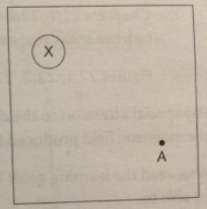# Problem: The diagram shows the cross section of wire carrying conventional positive current into the plane of the page. (You may ignore the earth's magnetic field)A) By means of an arrow on the diagram, show the direction in which a compass would point if placed at location A and describe the rule you use to remember this effect.B) Show the direction in which the compass would point at two other points of your own choosing.

###### FREE Expert Solution

Right-hand rule: The thumb points in the direction of the current while the fingers curl in the direction of the magnetic field.

The points into the page while the fingers curl in a clockwise direction.

84% (392 ratings)###### Problem Details

The diagram shows the cross section of wire carrying conventional positive current into the plane of the page. (You may ignore the earth's magnetic field)A) By means of an arrow on the diagram, show the direction in which a compass would point if placed at location A and describe the rule you use to remember this effect.

B) Show the direction in which the compass would point at two other points of your own choosing.

Frequently Asked Questions

What scientific concept do you need to know in order to solve this problem?

Our tutors have indicated that to solve this problem you will need to apply the Magnetic Field Produced by Straight Currents concept. You can view video lessons to learn Magnetic Field Produced by Straight Currents. Or if you need more Magnetic Field Produced by Straight Currents practice, you can also practice Magnetic Field Produced by Straight Currents practice problems.